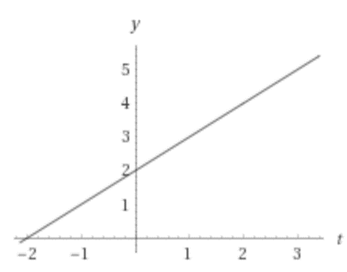# The domain and range of the function and sketch the graph.### Single Variable Calculus: Concepts...

4th Edition
James Stewart
Publisher: Cengage Learning
ISBN: 9781337687805### Single Variable Calculus: Concepts...

4th Edition
James Stewart
Publisher: Cengage Learning
ISBN: 9781337687805

#### Solutions

Chapter 1.1, Problem 38E
To determine

## To find: The domain and range of the function and sketch the graph.

Expert Solution

### Explanation of Solution

Given information:

The given function is,

H(t)=4t22t

Consider the given function,

H(t)=4t22t

The above equation is valid for all real number except,

2t0t2

Thus, the domain of the given function is all real numbers except t2 .

Consider the graph of the given function,The range of the given function is all real numbers except H(t)4.

### Have a homework question?

Subscribe to bartleby learn! Ask subject matter experts 30 homework questions each month. Plus, you’ll have access to millions of step-by-step textbook answers!